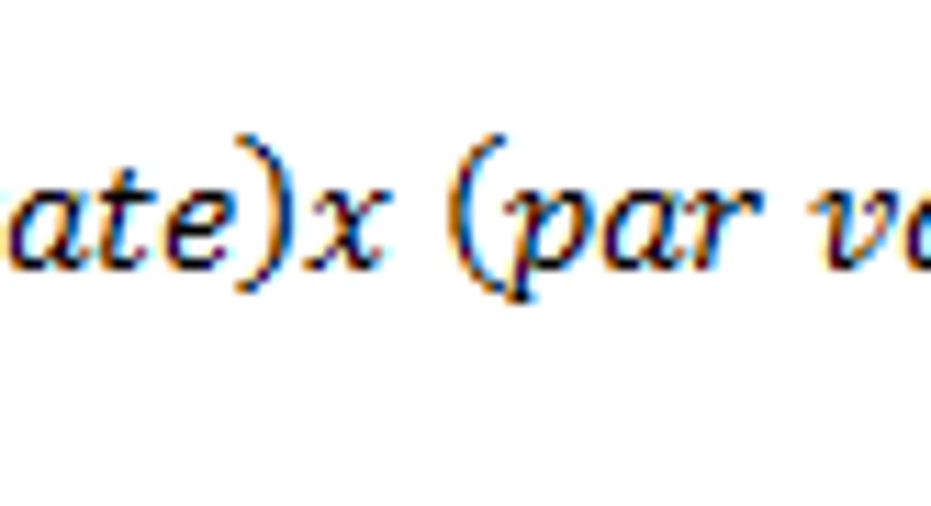# How to Calculate Dividend Distribution of Preferred StocksPhoto: 401kcalculator.org via Flickr.

Preferred stock is a special type of stock that trades on an exchange but works more like a bond than common stock. Like a bond, preferred stocks are bought primarily for their income potential and not for growth. Also as with a bond, preferred shareholders are ahead of common shareholders (but behind bondholders) in times of bankruptcy. Preferred stock can be a smart investment for income-seekers, and if you decide to invest, here's how to calculate the dividends you'll receive from your preferred stocks.

About preferred stock dividendsAnother similarity between preferred stocks and bonds is that while the market value of preferred shares can fluctuate, the dividends don't. Preferred stocks have a set dividend rate that's based on the "par value" of the stock -- usually \$25, but other amounts do exist. In other words, calculating preferred stock dividends is a fairly straightforward process, and you can expect the same dividend amount to continue, quarter after quarter and year after year.

Calculating your preferred stock dividend distributionYour preferred stock's dividend rate and par value can be found in the issuing company's preferred stock prospectus, so the first step is to locate this information.

Next, divide the dividend rate by 100 to convert it to a decimal for calculation purposes. For example, 5% becomes 0.05.

You can calculate your preferred stock's annual dividend distribution per share by multiplying the dividend rate and the par value.

If you want to determine how much your dividend will be on a quarterly basis (assuming your preferred stock pays quarterly), simply divide this result by four.

Finally, to determine the amount of money you'll receive, take the appropriate dividend (annual or quarterly) and multiply by the number of shares you own.

An exampleLet's say you just bought 100 shares of a preferred stock and want to know how much your quarterly dividend distributions will be. Upon reading the prospectus, you discover that the stock's par value is \$25 and its dividend rate is 6.5%.

Converting the dividend rate to a decimal produces 0.065, and multiplying by the \$25 par value gives us an annual dividend of \$1.625 per share. (Note: While you can't actually use a fraction of a cent, it's a good habit to hold off rounding your numbers until the end of a calculation.)

Dividing this result by four produces a quarterly dividend of \$0.40625 per share. Finally, multiplying by 100 shares gives a total quarterly dividend distribution of \$40.63, rounded to the nearest cent.